# combinatorics

(redirected from Combinational analysis)
Also found in: Dictionary.

## combinatorics

(kŏm'bənətôr`ĭks) or

## combinatorial analysis

(kŏm'bĭnətôr`ēəl), sometimes called the science of counting, the branch of mathematicsmathematics,
deductive study of numbers, geometry, and various abstract constructs, or structures; the latter often "abstract" the features common to several models derived from the empirical, or applied, sciences, although many emerge from purely mathematical or logical
concerned with the selection, arrangement, and operation of elements within sets. Combinatorial theory deals with existence (does a particular arrangement exist?), enumeration (how many such arrangements are there?) and structure (what are the properties of each arrangement?). It has applications in such diverse areas as managing computer and telecommunication networks, predicting poker hands, dividing tasks among workers, and finding a pair of socks in a drawer. Because combinatorics deals with concrete problems by limiting itself to finite collections of discrete objects, as opposed to the more common, continuous mathematics, it has neither standard algebraic manipulations nor a systematic problem-solving framework. Instead it relies upon the logical analysis of possibilities for each new problem, breaking the problem into a series of steps and substeps. Combinatorics has its roots in the 17th- and 18th-century attempts to analyze the odds of winning at games of chance. The advent of computers in the 20th cent. made possible the high-speed calculation required to analyze the multitude of possibilities inherent in a combinatorial approach to large-scale statistical testing and analysis. Branches of combinatorics include graph theory and combinations and permutations.

### Bibliography

See A. Slomson, An Introduction to Combinatorics (1991); A. Tucker, Applied Combinatorics (3d ed. 1994); R. A. Brualdi, Introductory Combinatorics (3d ed. 1997); M. Hall, Combinatorial Theory (2d ed. 1998); R. P. Stanley, Enumerative Combinatorics (1999).

The following article is from The Great Soviet Encyclopedia (1979). It might be outdated or ideologically biased.

## Combinatorics

(1) The same as mathematical combinatorial analysis.

(2) A branch of elementary mathematics concerned with the study of the number of combinations, obeying some condition or other, that may be formed out of a given finite set of objects (of any nature, for example, letters, numbers, arbitrary symbols).

The following are the most useful formulas of combinatorics.

(1) Number of arrangements. In how many ways can one select m objects from n different objects (taking into account the order in which the objects are selected)? The number of ways is equal to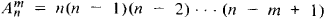Anm is said to be the number of arrangements of n elements taken m at a time.

(2) Number of permutations. Let us examine the following problem: in how many ways can one order n different objects? The number of ways is equal to

Pn = 1 · 2 · 3 . . . n = n!

(The symbol n! is read “n factorial”; it is also convenient to regard 0! as being equal to 1.) Pn is said to be the number of permutations of n elements.

(3) Number of combinations. In how many ways can one select m objects from n different objects (ignoring the order in which the objects are selected)? The number of ways is equal to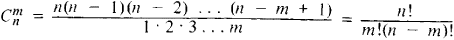Cnm is said to be the number of combinations of n elements taken m at a time. The numbers Cnm can be obtained as the coefficients in the expansion of the n th power of a binomial: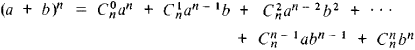Therefore they are also called binomial coefficients. Some basic relations of binomial coefficients are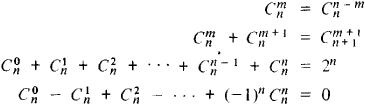The connection between the numbers Anm, Pm, and Cnm is given by the relation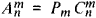Arrangements with repetition (that is, all possible choices of m objects of n different types, the order of choice being essential) and combinations with repetition (analogue of previous concept but without consideration of order) are also considered. The number of arrangements with repetition is nm, while the number of combinations with repetition is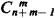Some fundamental rules for solving combinatorial problems follow.

(1) The sum rule. If a certain object A can be selected in m ways from a set of objects and if another object B can be selected in n ways, then there are m + n possibilities of choosing either A or B.

(2) The product rule. If an object A can be chosen in m ways and if after each such choice the object B can be chosen in n ways, then the ordered pair (A, B) can be chosen in mn ways.

(3) The principle of inclusion and exclusion. Let N objects be given possessing n properties α1, α2, . . . , αn. Let Ni, αj, . . . , αk) denote the number of objects possessing the properties αi, αj, . . . ,αk as well as perhaps other properties. Then the number N’ of objects that do not possess any of the properties α1, α2, . . . , αn is given by the formula

N’ = NN(∞1) – N(∞2) – . . . – N(∞n)

+ N(∞1, ∞2) + N(∞1, ∞3 + . . . + N(∞n – 1, ∞n)

N(∞1, ∞2, ∞3) – N(∞n – 2, ∞n – 1, ∞n

+ . . . + (–1)nN(∞1, . . . , ∞n

### REFERENCE

Netto, E. Lehrbuch der Combinatorik, 2nd ed. Leipzig-Berlin, 1927.

V. E. TARAKANOV